﻿ 基于非均匀网格有限差分法的大地电磁静位移模拟 Modeling of Magnetotelluric Static Shift Based on Non-Uniform Meshes Finite Difference Method

Vol. 08  No. 08 ( 2018 ), Article ID: 28150 , 9 pages
10.12677/AG.2018.88148

Modeling of Magnetotelluric Static Shift Based on Non-Uniform Meshes Finite Difference Method

Xiaokang Deng1,2, Xiaozhong Tong3*

1Hunan Provincial Key Laboratory of Hydropower Development Key Technology, Mid-South Design and Research Institute, Changsha Hunan

2School of Civil Engineering, Hunan University of Technology, Zhuzhou Hunan

3School of Geosciences and Info-Physics, Central South University, Changsha Hunan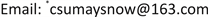Received: Dec. 3rd, 2018; accepted: Dec. 17th, 2018; published: Dec. 24th, 2018ABSTRACT

Because of the existence of shallow inhomogeneous bodies, static shift occurs in magnetotelluric sounding curves, which makes the interpretation of magnetotelluric data very difficult, or even impossible. It is very important to identify static shift in the interpretation of magnetotelluric data. In this paper, in order to compute the two-dimensional magnetotelluric responses of static shift model, non-uniform grids finite difference method was adopted for numerical results. From the electric field and magnetic field based on the differential equation, the linear equation for magnetotelluric numerical modeling was derived by non-uniform meshes finite difference method. By the numerical simulation for the geo-electric model with shallow inhomogeneous body, the character of static shift can be obtained and the magnetotelluric responses computed by non-uniform girds finite difference method are effective.

Keywords:Magnetotelluric, Two-Dimensional Model, Static Shift, Finite Difference Method, Non-Uniform Meshes

1中南勘测设计研究院水能资源利用关键技术湖南省重点实验室，湖南 长沙

2湖南工业大学土木工程学院，湖南 株洲

3中南大学地球科学与信息物理学院，湖南 长沙1. 引言

2. 非均匀网格差分正演算法

2.1. 边值问题

$\frac{\partial }{\partial y}\left(\frac{1}{\text{i}\omega \mu }\frac{\partial {E}_{x}}{\partial y}\right)+\frac{\partial }{\partial z}\left(\frac{1}{\text{i}\omega \mu }\frac{\partial {E}_{x}}{\partial z}\right)+\sigma {E}_{x}=0$ (1)

$\frac{\partial }{\partial y}\left(\frac{1}{\sigma }\frac{\partial {H}_{x}}{\partial y}\right)+\frac{\partial }{\partial z}\left(\frac{1}{\sigma }\frac{\partial {H}_{x}}{\partial z}\right)+\text{i}\omega \mu {H}_{x}=0$ (2)

$\nabla \cdot \left(\tau \nabla u\right)+\lambda u=0$ (3)

$u={E}_{x}$$\tau =\frac{1}{\text{i}\omega \mu }$$\lambda =\sigma$ (4)

$u={H}_{x}$$\tau =\frac{1}{\sigma }$$\lambda =\text{i}\omega \mu$ (5)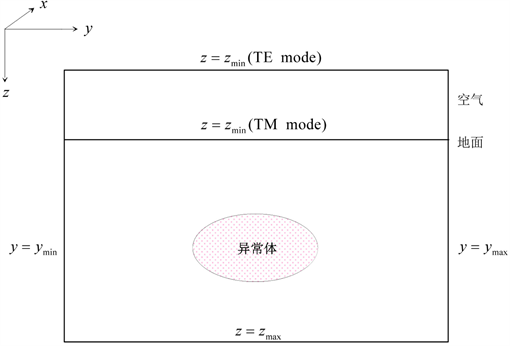Figure 1. Boundary conditions of 2D geo-electric model

1) TE极化模式的外边界条件

a) 上边界 $z={z}_{\mathrm{min}}$ 离地面足够远，使异常场在边界上为零，即以该处的 $u$ 为1单位

${u|}_{{z}_{\mathrm{min}}}=1$ (6)

b) 下边界 $z={z}_{\mathrm{max}}$ 为第三类边界条件，即

${\left(\frac{\partial u}{\partial z}+\sqrt{-\text{i}\omega \mu \sigma }u\right)|}_{z={z}_{\mathrm{max}}}=0$ (7)

c) 取左右边界 $y={y}_{\mathrm{min}}$$y={y}_{\mathrm{max}}$ 离局部不均匀体足够远，电磁场在 ${y}_{\mathrm{min}}$${y}_{\mathrm{max}}$ 上左右对称，其上的边界条件是

${\frac{\partial u}{\partial y}|}_{y={y}_{\mathrm{min}}}={\frac{\partial u}{\partial y}|}_{y={y}_{\mathrm{max}}}=0$ (8)

2) TM极化模式外边界条件

a) 上边界 $z={z}_{\mathrm{min}}$ 直接取在地面上，并以该处的 $u$ 为1单位，则有

${u|}_{z={z}_{\mathrm{min}}}=1$

2) 下边界 $z={z}_{\mathrm{max}}$ 的边界条件，同TE极化模式，见式(7)。

3) 左右边界 $y={y}_{\mathrm{min}}$$y={y}_{\mathrm{max}}$ 的边界条件，同TE极化模式，见式(8)。

2.2. 差分线性方程组导出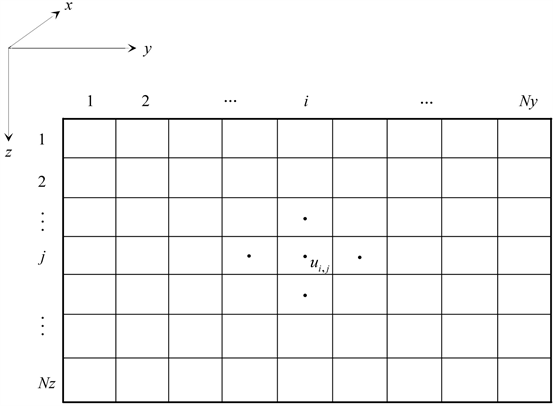Figure 2. Discretization of 2D geo-electric model

${\left[\frac{\partial }{\partial y}\left(\tau \frac{\partial u}{\partial y}\right)\right]}_{i,j}\approx \frac{1}{\Delta y}\left[{\left(\tau \frac{\partial u}{\partial y}\right)}_{i+\frac{1}{2},j}-{\left(\tau \frac{\partial u}{\partial y}\right)}_{i-\frac{1}{2},j}\right]\approx \frac{{\tau }_{i,j}+{\tau }_{i+1,j}}{2}\frac{{u}_{i+1,j}-{u}_{i,j}}{\Delta {y}^{2}}-\frac{{\tau }_{i,j}+{\tau }_{i-1,j}}{2}\frac{{u}_{i,j}-{u}_{i-1,j}}{\Delta {y}^{2}}$ (9)

${\left[\frac{\partial }{\partial z}\left(\tau \frac{\partial u}{\partial z}\right)\right]}_{i,j}\approx \frac{1}{\Delta z}\left[{\left(\tau \frac{\partial u}{\partial y}\right)}_{i,j+\frac{1}{2}}-{\left(\tau \frac{\partial u}{\partial y}\right)}_{i,j-\frac{1}{2}}\right]\approx \frac{{\tau }_{i,j}+{\tau }_{i,j+1}}{2}\frac{{u}_{i,j+1}-{u}_{i,j}}{\Delta {z}^{2}}-\frac{{\tau }_{i,j}+{\tau }_{i,j-1}}{2}\frac{{u}_{i,j}-{u}_{i,j-1}}{\Delta {z}^{2}}$ (10)

${\left[\frac{\partial }{\partial y}\left(\tau \frac{\partial u}{\partial y}\right)\right]}_{i,j}+{\left[\frac{\partial }{\partial z}\left(\tau \frac{\partial u}{\partial z}\right)\right]}_{i,j}+\lambda {u}_{i,j}=0$

$\left(\frac{{\tau }_{i,j}+{\tau }_{i+1,j}}{2}\frac{{u}_{i+1,j}-{u}_{i,j}}{\Delta {y}^{2}}-\frac{{\tau }_{i,j}+{\tau }_{i-1,j}}{2}\frac{{u}_{i,j}-{u}_{i-1,j}}{\Delta {y}^{2}}\right)+\left(\frac{{\tau }_{i,j}+{\tau }_{i,j+1}}{2}\frac{{u}_{i,j+1}-{u}_{i,j}}{\Delta {z}^{2}}-\frac{{\tau }_{i,j}+{\tau }_{i,j-1}}{2}\frac{{u}_{i,j}-{u}_{i,j-1}}{\Delta {z}^{2}}\right)+\lambda {u}_{i,j}=0$ (11)

$\begin{array}{l}\frac{{\tau }_{i,j}+{\tau }_{i+1,j}}{2\Delta {y}^{2}}{u}_{i+1,j}+\frac{{\tau }_{i,j}+{\tau }_{i-1,j}}{2\Delta {y}^{2}}{u}_{i-1,j}+\frac{{\tau }_{i,j}+{\tau }_{i,j+1}}{2\Delta {z}^{2}}{u}_{i,j+1}+\frac{{\tau }_{i,j}+{\tau }_{i,j-1}}{2\Delta {z}^{2}}{u}_{i,j-1}\\ \text{ }\text{ }\text{ }\text{ }\text{ }\text{ }\left(-\frac{{\tau }_{i-1,j}+2{\tau }_{i,j}+{\tau }_{i+1,j}}{2\Delta {y}^{2}}-\frac{{\tau }_{i,j-1}+2{\tau }_{i,j}+{\tau }_{i,j+1}}{2\Delta {z}^{2}}+\lambda \right){u}_{i,j}=0\end{array}$ (12)

$u=\left(\begin{array}{c}{u}_{1}\\ {u}_{2}\\ ⋮\\ \text{}{u}_{\left(i-1\right)×Nz+j}\\ {u}_{\left(i-1\right)×Nz+j+1}\\ ⋮\\ \text{}{u}_{Ny×{N}_{z}-1}\\ \text{}{u}_{Ny×{N}_{z}}\end{array}\right)=\left(\begin{array}{c}{u}_{1,1}\\ {u}_{1,2}\\ ⋮\\ {u}_{i,j}\\ {u}_{i,j+1}\\ ⋮\\ {u}_{Ny,{N}_{z}-1}\\ {u}_{Ny,{N}_{z}}\end{array}\right)$ (13)

$Ku=p$ (14)

2.3. 电磁响应计算

$\begin{array}{l}{Z}_{TE}={E}_{x}/\frac{1}{i\omega \mu }\frac{\partial {E}_{x}}{\partial z}\\ {\rho }_{a}^{TE}=\frac{1}{\omega \mu }{|{Z}_{TE}|}^{2}\\ {\phi }^{TE}=\mathrm{arctan}\frac{\mathrm{Im}\left[{Z}_{TE}\right]}{\mathrm{Re}\left[{Z}_{TE}\right]}\end{array}\right\}$ (15)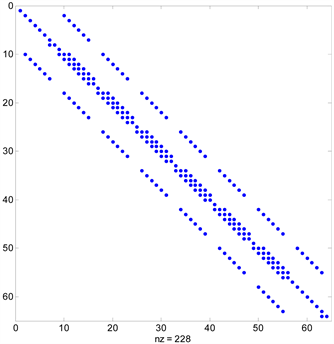Figure 3. The coefficient matrix of finite difference method for 2D magnetotelluric responses

$\begin{array}{l}{Z}_{TM}=-\frac{1}{\sigma }\frac{\partial {H}_{x}}{\partial z}/{H}_{x}\\ {\rho }_{a}^{TM}=\frac{1}{\omega \mu }{|{Z}_{TM}|}^{2}\\ {\phi }^{TM}=\mathrm{arctan}\frac{\mathrm{Im}\left[{Z}_{TM}\right]}{\mathrm{Re}\left[{Z}_{TM}\right]}\end{array}\right\}$ (16)

3. 静位移模型试算分析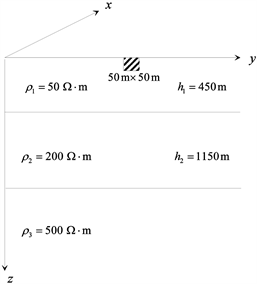Figure 4. 2D geo-electric model with static-shift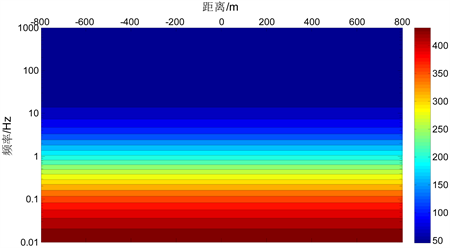(a) ${\rho }_{0}=50\text{}\Omega \cdot \text{m}$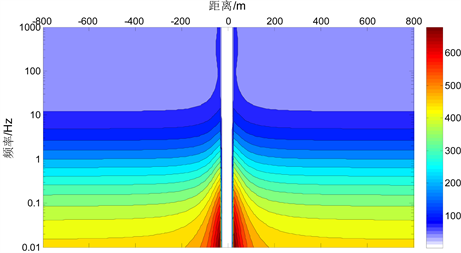(b) ${\rho }_{0}=5\text{}\Omega \cdot \text{m}$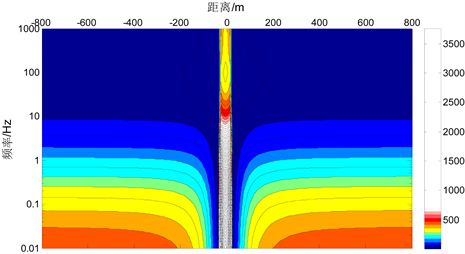(c) ${\rho }_{0}=500\text{}\Omega \cdot \text{m}$

Figure 5. Pseudo-section map of magnetotelluric responses of the uniform body in TM mode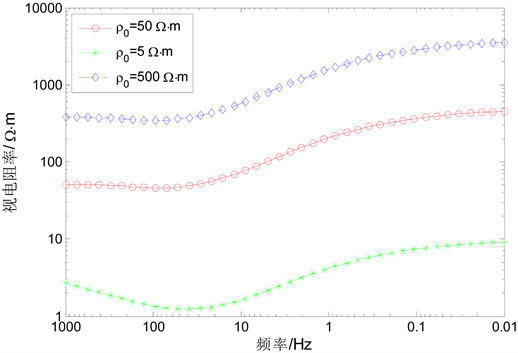Figure 6. The resistivity-frequency curves of the uniform body in TM mode

4. 结语

1) 针对各向同性介质中大地电磁场所满足的边值问题，详细推导了二维地电模型大地电磁场响应的有限差分正演算法；在保证计算精度的条件下，本文的非均匀网格剖分方式能减少正演计算时间，提高计算效率。

2) 通过对浅部不均匀体模型的模拟计算，得到了大地电磁的静位移响应规律，同时也验证了非均匀网格有限差分正演算法的准确性。

3) 高阻不均匀体使受影响测点的视电阻率整体向上移动而趋势不变，并且不均匀体电阻率越高，视电阻率向上平移的幅度越大。

4) 低阻不均匀体使受影响测点的视电阻率整体向下移动，并且由于电磁感应现象，单点视电阻率曲线在高频段趋势发生变化。

Modeling of Magnetotelluric Static Shift Based on Non-Uniform Meshes Finite Difference Method[J]. 地球科学前沿, 2018, 08(08): 1353-1361. https://doi.org/10.12677/AG.2018.88148

1. 1. 周邵民, 柳建新, 孙欢乐. 基于非负最小二乘法的一维MT正则化反演研究[J]. 工程地球物理学报, 2017, 14(3): 253-261.

2. 2. 伍亮, 李桐林, 朱成, 等. 大地电磁测深法中静态效应及其反演[J]. 地球物理进展, 2015, 30(2): 840-846.

3. 3. 李国瑞, 席振铢, 龙霞. 基于实测积累电荷静电场消除静态效应的方法[J]. 物探与化探, 2017, 41(4): 730-735.

4. 4. 陈辉, 王春庆, 孟小红, 等. 均匀大地中低阻静态模型及其静校正效果[J]. 工程地球物理学报, 2007, 4(4): 282-286.

5. 5. 冯德山，王珣. 大地电磁双二次插值FEM正演及最小二乘正则化联合反演[J]. 中国有色金属学报，2013，23(9)：2524-2531.

6. 6. 田红军, 佟铁钢. 带地形的二维各向异性大地电磁测深数值模拟[J]. 工程地球物理学报, 2015, 12(2): 139-144.

7. 7. 叶益信, 胡祥云, 何梅兴, 等. 电导率分块均匀大地电磁场二维有限元数值模拟[J]. 工程地球物理学报, 2009, 6(1): 9-14.

8. 8. de Groot-Hedlin, C. (2006) Finite-Difference Modeling of Magnetotelluric Fields: Error Estimates for Uniform and Nonuniform Grids. Geophysics, 71, 97-106. https://doi.org/10.1190/1.2195991

9. 9. Xiao, Q.B. and Zhao, G.Z. (2010) Comparison of Finite Difference Numerical Solutions in Magnetotelluric Modeling. Chinese Journal of Geophysics, 53, 622-630.

10. 10. Rao, K.P. and Babu, G.A. (2006) EMOD2D—A Program in C++ for Finite Difference Modeling of Magnetotelluric TM Mode Responses over 2D Earth. Computer & Geosciences, 32, 1499-1511. https://doi.org/10.1016/j.cageo.2006.02.017

11. 11. 王涛, 柳建新, 童孝忠, 等. 有限单元法与有限差分法在MT一维正演模拟中的对比分析[J]. 物探化探计算技术, 2013, 35(5): 538-543.

12. 12. Zhdanov, M. and Tolstaya, E. (2004) Minimum Support Nonlinear Parametrization in the Solution of a 3D Magnetotelluric Inverse Problem. Inverse Problem, 20, 937-952.

13. 13. Wannamaker, P.E. (1991) Advances in Three-Dimensional Magnetotelluric Modeling Using Integral Equations. Geophysics, 56, 1716-1728. https://doi.org/10.1190/1.1442984

14. 14. 张辉, 唐新功. 一种新型有限差分网格剖分方法在大地电磁一维正演中的应用[J]. 物探与化探, 2015, 39(3): 562-566.

15. 15. 柳建新, 童孝忠, 郭荣文, 等. 大地电磁测深法勘探[M]. 北京: 科学出版社, 2012.

16. NOTES

*通讯作者。